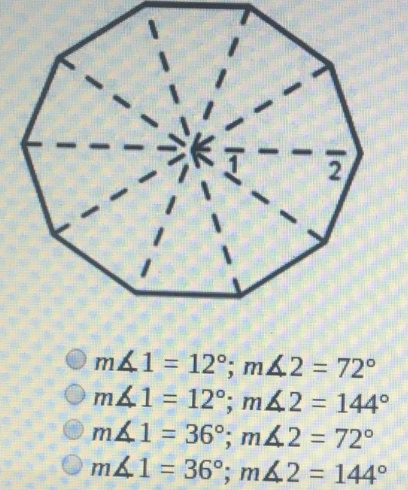# Given the regular polygon, what is the measure of each numbered angle?

Given the regular polygon, what is the measure of each numbered angle?